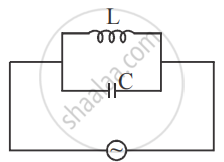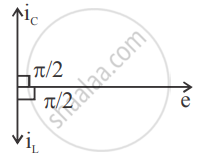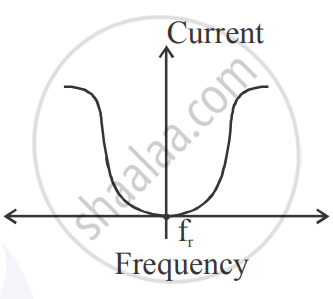Advertisement Remove all ads

# Assuming Expression for Impedance in a Parallel Resonant Circuit, State the Conditions for Parallel Resonance. Define Resonant Frequency and Obtain an Expression for It. - Physics

Sum

Assuming expression for impedance in a parallel resonant circuit, state the conditions for parallel resonance. Define resonant frequency and obtain an expression for it.

Advertisement Remove all ads

#### Solutioni=i_0 sin  ωt"i" ="i"_"c" - "i"_"L"

"e"/"z"="e"/"X"_"c"-"e"/"X"_"L"

1/"z"=1/"X"_"c"-1/"X"_"L"

For parallel resonance

"X"_"L"="X"_"C"

1/"z"=0

"z"= alpha

Resonant frequency (fr) :

The frequency at which impedance ( L )  because infinity and current becomes zero is called resonant frequency.

ω "L"= 1/ (ω "L")

ω ^2 "LC" =1

ω^2=1/("LC")

ω =1/sqrt("LC")

2pi "f"_"r" = 1/sqrt("LC")

"f"_"r" = 1/(2pi) 1/sqrt("LC")Is there an error in this question or solution?
Advertisement Remove all ads

#### APPEARS IN

Advertisement Remove all ads

#### Video TutorialsVIEW ALL 

Advertisement Remove all ads
Share
Notifications

View all notifications

Forgot password?